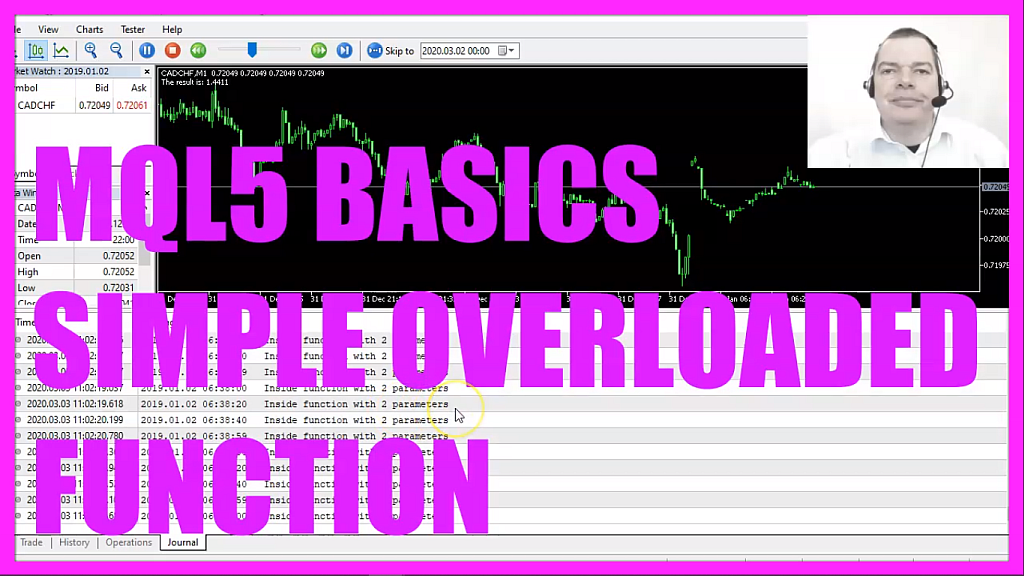In this video we are going to talk about function overloading, we have an output here that says we are inside a function with two parameters and we are going to create another function with the same name that will overload the first one, so let’s see how we can do that with mql5.
To get started please click on a little icon here or press F4 on your keyboard, now you should see the Metaeditor window and here you want to click on file, new file, expert advisor from template, continue, I will call this file simple function overloading, click on continue, continue and finish.
Now you can delete everything above the ontick function and the two comment lines here.
In this case, I would like to create a customized function called add values and I want to be able to add two or three values.
To have something to calculate we first want to get the ask price that is done by using symbol info double for the current symbol on the chart, I use symbol underscore ask and with normalize double and underscore digits we let Metatrader automatically calculate the right number of digits behind the dot.
Now let’s repeat the same for the bid price, this is equal but this time we are using symbol underscore bid and another variable name called bid and my third value will be the difference, that is simply a double value that is calculated and called difference and this is the ask price minus the bid price.
I would like to add the values for the ask and the bid price so I need to code a function that is called add values and this one takes the ask price and the bid price as a parameter.
So what does that mean?
Well, you might have seen other functions where you have to pass parameters like this one and when you remove the part behind the function name you will see that here is something that is called one of two and this is actually an overloaded function, we have two different ways to use it and that’s also what we want to do here in this example, I have passed two parameters, so let’s start with the first version of the overloaded function.
I will call that one add values it returns a double value and it takes two parameters, one for the ask value that we have passed here and the second one for the bid value that we have passed as a second parameter.
let’s use a print statement that will output inside function with two parameters in our journal.
Now comes a basic calculation where the return value will be equal to the ask value plus the bid value and finally we will return the calculated value to our main function here.
let’s add a comment statement here so we have an output on the chart saying the result is followed by the calculated result value and that’s it.
I can now compile the code but when I remove the parameters here I have only one version of my function, so far it is not overloaded so let’s create a new version, this is the same name of the function it’s the same return type but this one can take three parameters, once again we add the print statement but this time it will say inside function with three parameters, this time we calculate the return value by adding the first, the second and a third parameter and the rest is like in the function above.
Now let’s recompile the code and now when I remove the parameters here you can see that it is overloaded, we can either use the one that takes the ask value and the bid value or when we click on the overloaded description here we can also use the other one that will take the ask value the bid value and the difference value.
Well, if you don’t understand what all the code here does or if this was too fast for you maybe you want to watch the other videos in this basic video series first or maybe even the premium course on our website might be interesting for you. If you are already a premium course member and if you have a question like this that should be turned into a video, please send me an email, for now, we click on the compile button and if we don’t have any errors we can click the little button here to go back to Metatrader.
And in Metatrader, we click on view, strategy test or press control and r, please pick the new file simple function overloading dot ex5, mark the option for the visible mode here and start a test.
Here we see that we have a calculated return value and when we click on the journal tab we see that we are inside the function that will take two parameters, now let’s go back to Metaeditor and add the third parameter here, now I recompile the code, restart the test and this time it says that we are inside the function with three parameters.
Overloaded functions can be very helpful in complex systems and in this little video you have learned how to create an overloaded function for Metatrader 5 and you have coded it yourself with a few lines of mql5 code.